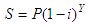# Salvage Value - how to calculate it with Matlab

This program calculates the salvage value of an item at the end of a given year. It is necessary for you to provide the age of the item, its original price, and its depreciation rate.

The value is obtained by the following formula:where:

S = salvage value
P = original price
i = nominal depreciation rate
Y = age in years

We show a video about how to use the formula. After the video, we show how to create a function to replicate the concept.

This is our simple Matlab code to calculate the above formula:

function sv = salvg_value(p, i, y)
i = i/100;
sv = p*(1-i)^y;

We create another script to test and drive the above m-file:

clc, clear, format compact, format bank

p = input('Enter original price: ');
i = input(
'Enter nominal depreciation rate: ');
y = input(
'Enter number of years: ');

sv = salvg_value(p, i, y)

Example 1:

What is the salvage value of Susan’s car if it is four years old, she bought it for \$4933.76, and it depreciates 21% annualy?

Now, let’s try our code...

Enter original price: 4933.76
Enter nominal depreciation rate: 21
Enter number of years: 4

The result is:

sv = 1921.70

Example 2:

Mary’s DVD player is 2 years old. What is its value if it cost \$155 originally and depreciates at a rate of 22%?

We launch our code...

Enter original price: 155
Enter nominal depreciation rate: 22
Enter number of years: 2

And the result is:

sv = 94.30

From 'Salvage Value' to home

From 'Salvage Value' to 'Finance Formulas'